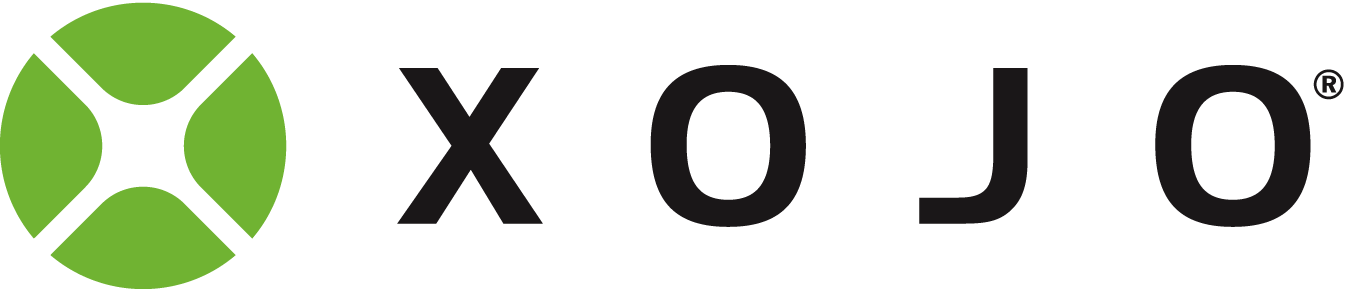# Plot A to B

If I want to draw a line (or plot a series of points along the line) A to B and then erase it what would the best method be?

Thanks Emile

So if I have the start point and end point I would just add some extra in between points and the change colour to the background repeat to clear??

Var points() As Integer
points = Array(10, 10, 100, 50, 10, 200, 10, 10)
g.DrawingColor = RGB(100, 200, 255)
g.FillPath(points)

Strange, I was thinking you wanted to draw a line…

Thanks Mike, I should have defined the problem better. I can draw a line.
How would you plot a series of points in a straight line? I can’t see plotpoint?

1 Like

What about using iOSPath? iOSPath can be open or closed and can even be used to produce composite objects (e.g., donut-like shapes with holes in the middle).

Move discussion to Calculate Endpoint from Bearing